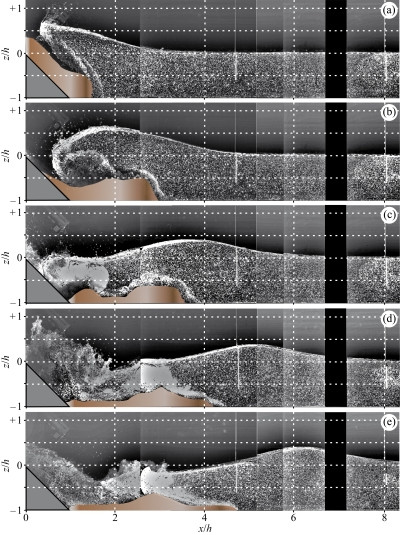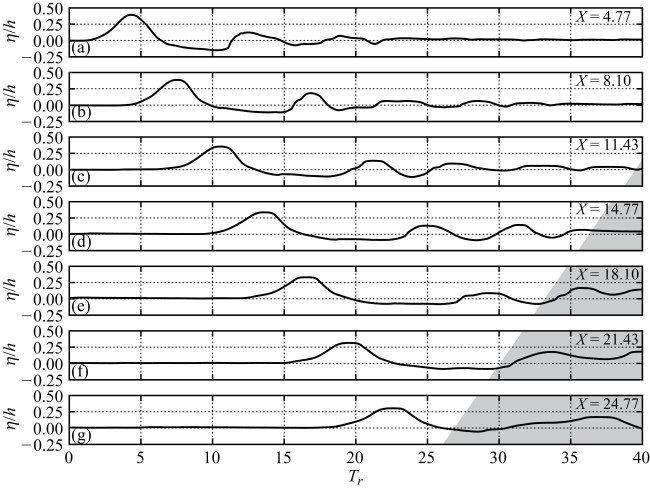## Test 07

Subaerial landslide generated impulse waves in a wave channel

(V. Heller)

(Download full test case data files here: SPHERIC_TestCase7.zip)

### Introduction

Impulse waves in oceans, bays, lakes, or reservoirs are generated by landslides, rock falls, shore instabilities, snow avalanches, glacier calvings, or meteorite impacts. Examples are the 1958 Lituya Bay case in Alaska where the generated impulse wave reached a maximum run-up height of 524 m on the opposite shore or the 1963 Vaiont case in North Italy where an impulse wave overtopped a dam by about 70 m and killed 2,000 people. The mainly passive methods to face such catastrophes include evacuations, water level draw-down, or freeboard control in artificial reservoirs. They require detailed knowledge of the wave features and of the wave effects on the dam or shore line. Numerical methods such as SPH may play an important role in the future in predicting the effects of impulse waves since numerical models may result in more accurate predictions for complex geometries than general physical model studies at lower costs than specific physical case studies (Heller et al. 2009). This test case is one out of three experiments presented in Heller (2007), conducted in a wave channel with a still water depth h = 0.300 m. The results include the granular slide deformation prior and during impact into the water body, the wave generation including the temporal advance of velocity vector fields measured with Particle Image Velocimetry PIV, and the wave profiles measured with seven Capacitance Wave Gages CWGs.

### Flow phenomena

Two-dimensional granular slide deformation on a slope and during impact into a water body; Two-dimensional impulse wave generation and propagation; Unsteady three phase flow including granulate, water, and air.

### Geometry

Figure 1 shows a definition sketch of the relevant parameters, with the specific values for this benchmark test case listed in Table 1. The slide consists of cylindrical shaped granular slide material with a grain diameter dg = 4 mm, an internal friction angle phi = 34°, a grain density ρg = 2,745 kg/m3, and a bulk slide density ρs = ρg(1 − n) = 1,678 kg/m3 with a bulk slide porosity n = 38.9%. Note that the bulk slide volume Vs was set identical to the filled inner slide box volume (Section “Boundary conditions”) and the corresponding bulk slide density ρs = ms / Vs was also calculated with Vs and the slide mass ms. The parameters n, Vs, and ρs may therefore be larger during slide impact than specified in Table 1. The parameter Vs is the slide centroid impact velocity and s the maximum slide thickness during slide impact calculated or measured, respectively, at Laser Distance Sensor LDS0 in Figure 3. The water temperature was 23°C. The dimensionless parameters are listed below in Table 1 and include the slide Froude number F = Vs / (gh)1 / 2 = 2.72 with the gravitational acceleration g, relative slide thickness S = s/h = 0.32, relative slide mass M = ms / (ρwbh2) = 1.25 with the water density ρw, and the slide impact angle α = 45°.Figure 1. Definition GeometryTable 1. Relevant dimensional and dimensionless parametersFigure 2. Positioning of the seven capacitance wave gages along channel axisFigure 3. Dawn of slide kinematics and positioning of LDSs Boundary conditions

### Boundary conditions

A rectangular slide box was filled with granular slide material and accelerated with a pneumatic landslide generator from the initial centroid position at −2.200 m above the coordinate origin along the hill slope ramp. This pneumatic landslide generator allowed a high test repetition accuracy (Section “Repeatability”). At −1.285 m above the coordinate origin in the ramp direction, the slide box reached the maximum velocity of Vbox = 3.25 m/s and its front flap opened. The box velocity was determined from the measurements of a cable actuated position sensor which recorded the box position as a function of time. The granular slide material left the slide box and accelerated further down the ramp to generate impulse waves in the wave channel. The slide impact velocity Vs was determined with the energy balance between the slide release position and LDS−1 and between LDS−1 and the slide impact location LDS0, taking into account the dynamic bed friction angle δ between the slide and the slope ramp.

### Initial conditions

Slide shape modelling: The granular slide of dimensions 0.600 m × 0.118 m × 0.472 m left the slide box with the velocity Vbox = 3.25 m/s and propagated thereafter free on the hill slope ramp (Figure 3). Note that the width of the slide box is only 94% of the channel width b = 0.500 m and that there is a gap of 14 mm between the inner slide box bottom and the hill slope ramp. The slide properties are listed in Table 1.

Velocity vector fields or wave profiles modelling: The slide shape during impact is shown in Figure 4 and the channel geometry is reported in the Section “Geometry”.Figure 4. Slide profiles vs time

### Fluid Properties

The fluid is incompressible and Newtonian.

### Discretisation

The slide consists of grains with a diameter of dg = 4 mm. One velocity vector in Figure 6 represents an interrogation window size of 49.7(L) mm × 24.9(H) mm whereas in the ASCII files the resolution is 12.4(L) mm × 12.4(H) mm.

### Results specification

A simulation may include the granular slide profiles at LDS−1 (Figure 4), at LDS0, and also at the deposit position in the water body (Figure 5(e)). Further, the velocity vector fields in the slide impact zone and the free surface may be compared with Figure 6 and the data in the corresponding ASCII files. The relative wave profiles may be simulated and compared with the wave profiles shown in Figure 7 or the data in the corresponding ASCII files.Figure 5. Raw PIV Images & Figure 6. Velocity vector fields

### Rules Format

The velocity vector fields are available for the five time steps in Figure 6 in the ASCII files “Vectors Figure 6.txt” of dimensions 12,288 × 4 with column 1 = x (mm) coordinate, column 2 = z (mm) coordinate, column 3 = x (m/s) velocity components, and column 4 = z (m/s) velocity components with a resolution of 12.4(L) mm × 12.4(H) mm. Note that no vectors are available in the region of the black vertical steel profile (Figure 5). The water profiles or masks of the images shown in Figure 6 for each time step are also available in the ASCII files “Mask Figure 6.txt” with column 1 = x (mm) coordinate and column 2 = z (mm) coordinate. The ASCII file “Slides Figure 4 and wave profiles Figure 7.txt” includes both the slide and wave profiles with column 1 = t (s) time, column 2 = ξ (mm) coordinate of the slide at LDS−1 (Figure 4), column 3 = ξ (mm) coordinate of the slide at LDS0, column 4 = η (mm) water surface profile at CWG1 (Figure 7), column 5 = η (mm) water surface profile at CWG2, column 6 = η (mm) water surface profile at CWG3, column 7 = η (mm) water surface profile at CWG4, column 8 = η (mm) water surface profile at CWG5, column 9 = η (mm) water surface profile at CWG6, and column 10 = η (mm) water surface profile at CWG7.Figure 7. Relative wave profiles η/h versus relative time Tr = t(g / h)1 / 2 at (a) CWG1, (b) CWG2, (c) CWG3, (d) CWG4, (e) CWG5, (f) CWG6, and (g) CWG7; the grey areas are influenced by wave reflection

### Benchmark results

Figure 4 shows the slide profiles ξ(t) versus time t at LDS-1 (grey) and during impact at LDS0 (black). The slide profile in the end position on the channel bottom is available from Figures 5(e) or 6(e), respectively. Figure 5 shows the PIV raw images from the slide impact zone in the (x/h, z/h) plane corresponding to an area of 2.5 m × 0.7 m disturbed by a black vertical steel profile of the wave channel. The granular slide material marked in brown dives into the water body in Figure 5(a) developing flow separation between the slide surface and the water body at relative time Tr = t(g / h)1 / 2 = 1.14. The slide progresses further into the water body while the water above the slide starts to collapse in Figure 5(b). The impact crater collapses completely on the top of the slide material in Figure 5(c) and produces an air-water phase mixing. The crest of the primary wave passes CWG1 (white vertical rod) in Figure 5(d) and the measured wave profile is available from Figure 7(a). The slide material reaches its final position in Figure 5(e). A part of the flow runs up the hill slope ramp to generate a secondary, bore-type impulse wave not shown herein. The velocity vector fields for the area in Figure 5 are shown in Figure 6. One velocity vector represents a rectangle of 49.7(H) mm × 24.9(V) mm whereas the resolution in the corresponding ASCII files is larger (Section “Discretisation”). Figure 6(a) shows the water displacement to the right and to the top with maximum velocity vectors of about 1.5 times the reference vector (gh)1 / 2 = 1.72 m/s next to the slide front, but smaller than the slide impact velocity Vs = 4.67 m/s. The impact crater collapse in the vertical direction is apparent from Figure 6(b). Within the extreme air-water mixture of Figure 6(c) the PIV system fails to result in well-defined velocity vectors. The velocity vectors indicate a flow direction up the hill slope ramp in Figure 6(d). The run-up water particle velocities are small in Figure 6(e) initiating the run-down phase. Figure 7 relates to the relative wave profiles η/h versus the relative time Tr = t(g / h)1 / 2. Figure 7 shows from top (a) to bottom (g) the data at CWG1 to CWG7. In Figure 7(a) at CWG1 the primary impulse wave has a relative wave amplitude a/h = 0.39 and a relative wave trough amplitude at a/h = 0.15. The impulse wave undergoes a wave height decay and a dispersion process from Figure 7(a) to (g). In Figure 7(g) the relative wave amplitude is a/h = 0.30 and the wave amplitude is decreased by 23% between CWG1 and CWG7. The grey zones in Figures 7(c) to (g) refer to wave data affected by wave reflection at the wave absorber (Figure 2).

### Repeatability

The images in Figures 5 and 6, respectively, consist of two tests with identical test parameters. The following statements about the repeatability of the presented test are based on the comparison of the measurements of these two “identical” experiments. The maximum slide run-out distance is one relevant parameter to control the repeatability of the slide profile. It was measured at 1.42 m in the first, and 1.39 m (–2.1%) in the repeated test. The wave amplitude at the seven CWGs between these two tests deviate 1.3% to 7.9% where their points in time differ maximal 2%. These deviations are also apparent in Figure 5(e) at relative distance x/h = 5.16 where the water surface of the two tests match not perfectly. This comparison shows the high test repeatability of this three phase phenomenon which is due to the control of the slide kinematics with the pneumatic landslide generator (Heller 2007).

### Suggestions

If you have something to add or if there is something else you think should be added, please write me to v.heller@ soton.ac.uk.

### References

Heller, V. (2007). Landslide generated impulse waves - Prediction of near field characteristics. Dissertation 17531, ETH Zurich, Zurich. Online: http://e-collection.ethbib.ethz.ch/view/eth:30012

Heller, V., Hager, W.H., Minor, H.-E. (2009). Landslide generated impulse waves in reservoirs - Basics and computation. VAW Mitteilung 211, Boes, R. (ed.), ETH Zurich, Zurich. Online: http://www.vaw.ethz.ch/news/newest_vaw_reports/index_EN